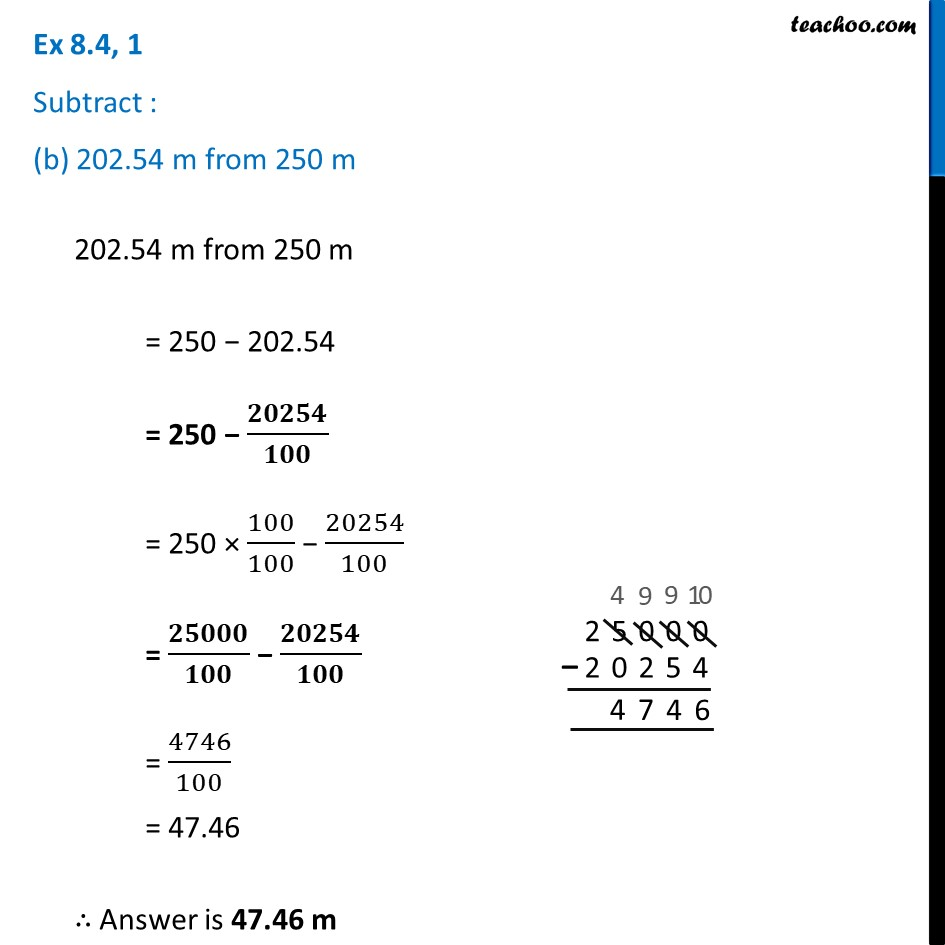Ex 8.4

Chapter 8 Class 6 Decimals
Serial order wiseLearn in your speed, with individual attention - Teachoo Maths 1-on-1 Class

### Transcript

Ex 8.4, 1 Subtract : (b) 202.54 m from 250 m202.54 m from 250 m = 250 − 202.54 = 250 − 𝟐𝟎𝟐𝟓𝟒/𝟏𝟎𝟎 = 250 × 100/100 − 20254/100 = 𝟐𝟓𝟎𝟎𝟎/𝟏𝟎𝟎 − 𝟐𝟎𝟐𝟓𝟒/𝟏𝟎𝟎 = 4746/100 = 47.46 ∴ Answer is 47.46 m